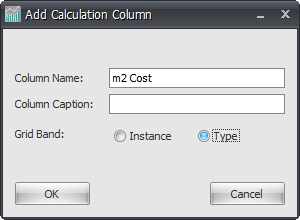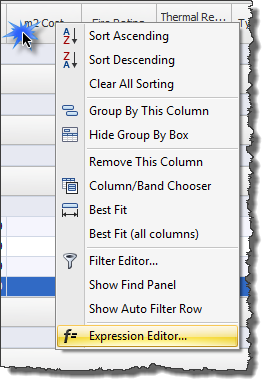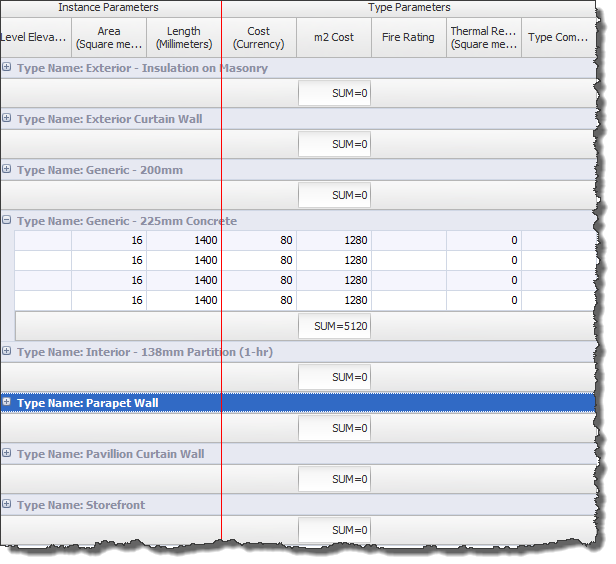Wikis > RTV Reporter – Interface Overview > RTV Reporter Add Calculation Column

With Reporter you have the ability to create your own custom Calculation column using the actual Revit parameter values from your project.

Examples of this could be when generating costing reports and you may want to combine values for say m2 x \$ value to give you the calculated value as a result.

If we use the Walls Category example below you can see that there are values for the Area and a Cost.  You could create a custom Calculation Column combining these two figures together.Click the Add Calculation Column button from the ribbon.Enter the required Column Name and select either Instance or Type before clicking OK.Once the column is created you can of course drag the column header to position it where required.  You can then right click on the column header and select Expression Editor to then define the required Calculation for the column.In The Expression Editor box you can define you own custom calculation formula as required using any of the options available.  In this example we simply want to multiply the two Revit Columns (Fields) together so therefore select the Fields option in the left hand window to display the available fields in the right hand window.  Double click the required fields to be used in the formula which then adds them to the top window as shown below and between them add the ‘multiply’ option between the two fields.You will then see the Calculation column automatically populates as shown below.In addition to this you can then get a sub total on the column.  To do so right click in the column footer and select Sum from the pop-up menu.You will then see the sub totals appear in each of the footer rows in the Calculated Column as shown below.  Note: You can do this on any column not just a Calculated Column.Category: